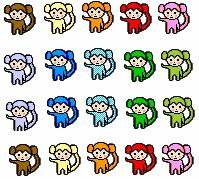Definition Of Multiplication

Multiplication of two numbers is the repeated addition of one number to the number of times equal to the other number.

Multiplication is one of the basic arithmetic operations.
The multiplication symbol is denoted by ×.
Multiplication of numbers is commutative, associative, and distributive.

Examples of Multiplication

4 × 5 = 20To multiply 4 with 5, 4 can be added 5 times i.e. 4 + 4 + 4 + 4 + 4 = 20.

Solved Example on Multiplication

Ques: Cathy distributed 3 apples each to 6 of her friends. The number of apples she had in all, is given by 3 × 6. Identify the repeated addition statement that represents 3 × 6.

Choices:

A. 4 + 4 + 4 + 4
B. 9 + 9 + 9
C. 3 + 3 + 3 + 3 + 3 + 3
D. 6 + 6 + 6 + 6